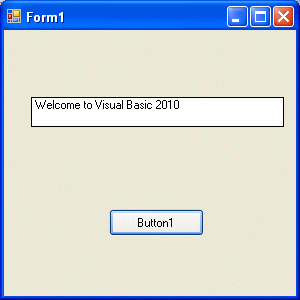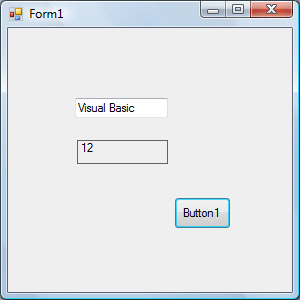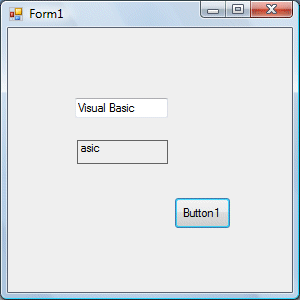# Visual Basic 2010 Lesson 8- String Manipulation

#### [Lesson 7] << [CONTENTS] >> [Lesson 9]

Generally, we have to deal with two types of data, the numeric and the non-numeric data types. The non-numeric data again divided into a few types, the most common one being text, or string. Examples of the string are words, sentences, names, addresses, gender, cities, book titles and much more. In this lesson, we shall learn how to process and manipulate strings in visual basic 2010

### 8.1 String Manipulation Using + and & signs.

In Visual Basic 2010, Strings can be manipulated using the & sign and the + sign, both perform the string concatenation which by combining two or more smaller strings into larger strings. For example, we can join “Visual” and “Basic” into “Visual Basic” using “Visual”&”Basic” or “Visual “+”Basic”, as shown in the example below

#### Example 8.1(a)

```Private Sub Button1_Click(ByVal sender As System.Object, ByVal e As System.EventArgs) Handles Button1.Click
Dim text1, text2, text3 As String
text1 = "Visual"
text2 = "Basic"
text3 = text1 + text2
Label1.Text = text3
End Sub
```

#### Example 8.1(b)

```Dim text1, text3 as string
Dim Text2 As Integertext1 = "Visual"
text2=22
text3=text1+text2
Label1.Text = text3
```

This code will produce an error because of data mismatch.However, using & instead of + will be all right.

```Dim text1, text3 as string
Dim Text2 As Integer
text1 = "Visual"
text2=22
text3=text1 & text2
Label1.Text = text3
```

You can combine more than two strings to form a larger string, like the following example:

```Public Class Form1
Private Sub Button1_Click(ByVal sender As System.Object, ByVal e As System.EventArgs) Handles Button1.Click
Dim text1, text2, text3, text4, text5, text6 As String
text1 = "Welcome"
text2 = " to"
text3 = " Visual"
text4 = " Basic"
text5 = " 2010"
text6 = text1 + text2 + text3+text4+text5
Label1.Text = text6
End Sub
End Class
```

Running the above program will produce the following screen shot.### 8.2 String Manipulation Using Visual Basic 2010 Built-in Functions

A function is similar to a normal procedure. However, the main purpose of a function is to accept an input and return a value. The value then is passed on to the main program to finish the in this lesson here and will explain the rest of them in later lessons.

#### 8.2 (a) The Len Function

The length function returns an integer value equals to the length of a phrase or a sentence, including the empty spaces. The syntax is

```Len ("Phrase")
```

For example,

`Len (Visual Basic) = 12`

and

` Len (welcome to VB tutorial) = 22`

#### Example 8.3

```Public Class Form1
Private Sub Button1_Click(ByVal sender As System.Object, ByVal e As System.EventArgs) Handles Button1.Click
Label1.Text = Len(TextBox1.Text)
End Sub
End Class
```

The output:8.2(b) The Right Function

The Right function extracts the right portion of a phrase. The syntax for Visual Basic 6 is

```Right ("Phrase", n)
```

Where n is the starting position from the right of the phase where the portion of the phrase is going to be extracted. For example,

```Right("Visual Basic", 4) = asic
```

However, this syntax is not applicable in VB2010. In VB2010, we need use the following syntax

```Microsoft.VisualBasic.Right("Phrase",n)
```

#### Example 8.2(a)

```Private Sub Button1_Click(ByVal sender As System.Object, ByVal e As System.EventArgs) Handles Button1.Click
Dim text1 As String
text1 = TextBox1.Text
Label1.Text = Microsoft.VisualBasic.Right(text1, 4)
End Sub
```

The above program will return four rightmost characters of the phrase entered into the textbox.

The Output:*The reason of using the full reference is because many objects have the Right properties so using Right on its own will make it ambiguous to VB2010.

#### 8.2(c)The Left Function

The Left function extracts the left portion of a phrase. The syntax is

```Microsoft.VisualBasic.Left("Phrase",n)
```

Where n is the starting position from the left of the phase where the portion of the phrase is going to be extracted. For example,

```Microsoft.VisualBasic.Left ("Visual Basic", 4) = Visu .
```

[Lesson 7] << [CONTENTS] >> [Lesson 9]